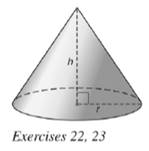Chapter 9.3, Problem 23EElementary Geometry For College St...

7th Edition
Alexander + 2 others
ISBN: 9781337614085

Solutions

Chapter
SectionElementary Geometry For College St...

7th Edition
Alexander + 2 others
ISBN: 9781337614085
Textbook Problem

For the right circular cone shown in Exercise 22, suppose that h = 7 in. and r = 6 in. Find the exact and approximatea) lateral area.b) total area.c) volume.To determine

(a)

To find: The exact and approximate lateral area of the right circular cone.

Explanation

Given:

Consider a right circular cone with h=7 in and r=6 in.

Formula used:

In a right circular cone r2+h2=l2.

The lateral area of the right circular cone is L=πrl

Calculation:

We have h=7 in and r=6 in.

Substitute the known values in the formula,

r2+h2=l262+72=l236+49

To determine

(b)

To find: The exact and approximate total area of the right circular cone.

To determine

(c)

To find: The exact and approximate volume of the right circular cone.

Still sussing out bartleby?

Check out a sample textbook solution.

See a sample solution

The Solution to Your Study Problems

Bartleby provides explanations to thousands of textbook problems written by our experts, many with advanced degrees!

Get Started